# Resources tagged with: Similarity and congruence

Filter by: Content type:
Age range:
Challenge level:

### There are 51 results

Broad Topics > Angles, Polygons, and Geometrical Proof > Similarity and congruence### Partly Circles

##### Age 14 to 16 Challenge Level:

What is the same and what is different about these circle questions? What connections can you make?### Strange Rectangle

##### Age 16 to 18 Challenge Level:

ABCD is a rectangle and P, Q, R and S are moveable points on the edges dividing the edges in certain ratios. Strangely PQRS is always a cyclic quadrilateral and you can find the angles.### Napkin

##### Age 14 to 16 Challenge Level:

A napkin is folded so that a corner coincides with the midpoint of an opposite edge . Investigate the three triangles formed .### Fitting In

##### Age 14 to 16 Challenge Level:

The largest square which fits into a circle is ABCD and EFGH is a square with G and H on the line CD and E and F on the circumference of the circle. Show that AB = 5EF. Similarly the largest. . . .### Arrh!

##### Age 14 to 16 Challenge Level:

Triangle ABC is equilateral. D, the midpoint of BC, is the centre of the semi-circle whose radius is R which touches AB and AC, as well as a smaller circle with radius r which also touches AB and AC. . . .### Sitting Pretty

##### Age 14 to 16 Challenge Level:

A circle of radius r touches two sides of a right angled triangle, sides x and y, and has its centre on the hypotenuse. Can you prove the formula linking x, y and r?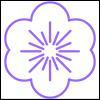### Flower

##### Age 16 to 18 Challenge Level:

Six circles around a central circle make a flower. Watch the flower as you change the radii in this circle packing. Prove that with the given ratios of the radii the petals touch and fit perfectly.### All Tied Up

##### Age 14 to 16 Challenge Level:

A ribbon runs around a box so that it makes a complete loop with two parallel pieces of ribbon on the top. How long will the ribbon be?### Chord

##### Age 16 to 18 Challenge Level:

Equal touching circles have centres on a line. From a point of this line on a circle, a tangent is drawn to the farthest circle. Find the lengths of chords where the line cuts the other circles.### Nicely Similar

##### Age 14 to 16 Challenge Level:

If the hypotenuse (base) length is 100cm and if an extra line splits the base into 36cm and 64cm parts, what were the side lengths for the original right-angled triangle?### Triangle Midpoints

##### Age 14 to 16 Challenge Level:

You are only given the three midpoints of the sides of a triangle. How can you construct the original triangle?### From All Corners

##### Age 14 to 16 Challenge Level:

Straight lines are drawn from each corner of a square to the mid points of the opposite sides. Express the area of the octagon that is formed at the centre as a fraction of the area of the square.### Pent

##### Age 14 to 18 Challenge Level:

The diagram shows a regular pentagon with sides of unit length. Find all the angles in the diagram. Prove that the quadrilateral shown in red is a rhombus.### Pythagoras Proofs

##### Age 14 to 16 Challenge Level:

Can you make sense of these three proofs of Pythagoras' Theorem?### Trapezium Four

##### Age 14 to 16 Challenge Level:

The diagonals of a trapezium divide it into four parts. Can you create a trapezium where three of those parts are equal in area?### Figure of Eight

##### Age 14 to 16 Challenge Level:

On a nine-point pegboard a band is stretched over 4 pegs in a "figure of 8" arrangement. How many different "figure of 8" arrangements can be made ?### The Line and Its Strange Pair

##### Age 14 to 16 Challenge Level:

In the diagram the point P' can move to different places along the dotted line. Each position P' takes will fix a corresponding position for P. If P' moves along a straight line what does P do ?### Mapping the Wandering Circle

##### Age 14 to 16 Challenge Level:

In the diagram the point P can move to different places around the dotted circle. Each position P takes will fix a corresponding position for P'. As P moves around on that circle what will P' do?### Two Triangles in a Square

##### Age 14 to 16 Challenge Level:

Given that ABCD is a square, M is the mid point of AD and CP is perpendicular to MB with P on MB, prove DP = DC.### Slippage

##### Age 14 to 16 Challenge Level:

A ladder 3m long rests against a wall with one end a short distance from its base. Between the wall and the base of a ladder is a garden storage box 1m tall and 1m high. What is the maximum distance. . . .##### Age 14 to 16 Challenge Level:

Find the area of the shaded region created by the two overlapping triangles in terms of a and b?### Triangle in a Triangle

##### Age 14 to 16 Challenge Level:

Can you work out the fraction of the original triangle that is covered by the inner triangle?### Wedge on Wedge

##### Age 14 to 16 Challenge Level:

Two right-angled triangles are connected together as part of a structure. An object is dropped from the top of the green triangle where does it pass the base of the blue triangle?### Making Sixty

##### Age 14 to 16 Challenge Level:

Why does this fold create an angle of sixty degrees?### Points in Pairs

##### Age 14 to 16 Challenge Level:

Move the point P to see how P' moves. Then use your insights to calculate a missing length.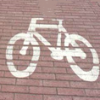### It Depends on Your Point of View!

##### Age 14 to 16 Challenge Level:

Anamorphic art is used to create intriguing illusions - can you work out how it is done?### Kite in a Square

##### Age 14 to 16 Challenge Level:

Can you make sense of the three methods to work out the area of the kite in the square?##### Age 14 to 16 Challenge Level:

Two ladders are propped up against facing walls. The end of the first ladder is 10 metres above the foot of the first wall. The end of the second ladder is 5 metres above the foot of the second. . . .### A Sameness Surely

##### Age 14 to 16 Challenge Level:

Triangle ABC has a right angle at C. ACRS and CBPQ are squares. ST and PU are perpendicular to AB produced. Show that ST + PU = AB### Doubly Isosceles

##### Age 14 to 16 Short Challenge Level:

Find the missing distance in this diagram with two isosceles triangles### Matter of Scale

##### Age 14 to 16 Challenge Level:

Prove Pythagoras' Theorem using enlargements and scale factors.### Look Before You Leap

##### Age 14 to 16 Challenge Level:

Can you spot a cunning way to work out the missing length?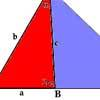### Double Angle Triples

##### Age 16 to 18 Challenge Level:

Try out this geometry problem involving trigonometry and number theory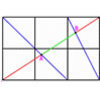##### Age 16 to 18 Challenge Level:

A new problem posed by Lyndon Baker who has devised many NRICH problems over the years.### Parallel Universe

##### Age 14 to 16 Challenge Level:

An equilateral triangle is constructed on BC. A line QD is drawn, where Q is the midpoint of AC. Prove that AB // QD.### Pentakite

##### Age 14 to 18 Challenge Level:

ABCDE is a regular pentagon of side length one unit. BC produced meets ED produced at F. Show that triangle CDF is congruent to triangle EDB. Find the length of BE.### Golden Triangle

##### Age 16 to 18 Challenge Level:

Three triangles ABC, CBD and ABD (where D is a point on AC) are all isosceles. Find all the angles. Prove that the ratio of AB to BC is equal to the golden ratio.### Angle Trisection

##### Age 14 to 16 Challenge Level:

It is impossible to trisect an angle using only ruler and compasses but it can be done using a carpenter's square.### Fit for Photocopying

##### Age 14 to 16 Challenge Level:

Explore the relationships between different paper sizes.### Folding Squares

##### Age 14 to 16 Challenge Level:

The diagonal of a square intersects the line joining one of the unused corners to the midpoint of the opposite side. What do you notice about the line segments produced?### Pentabuild

##### Age 16 to 18 Challenge Level:

Explain how to construct a regular pentagon accurately using a straight edge and compass.### Same Length

##### Age 11 to 16 Challenge Level:

Construct two equilateral triangles on a straight line. There are two lengths that look the same - can you prove it?### Folding Fractions

##### Age 14 to 16 Challenge Level:

What fractions can you divide the diagonal of a square into by simple folding?##### Age 14 to 18 Challenge Level:

How would you design the tiering of seats in a stadium so that all spectators have a good view?### Squirty

##### Age 14 to 16 Challenge Level:

Using a ruler, pencil and compasses only, it is possible to construct a square inside any triangle so that all four vertices touch the sides of the triangle.### Triangular Slope

##### Age 14 to 16 Short Challenge Level:

Can you find the gradients of the lines that form a triangle?### Another Triangle in a Triangle

##### Age 16 to 18 Challenge Level:

Can you work out the fraction of the original triangle that is covered by the green triangle?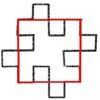### Squareflake

##### Age 16 to 18 Challenge Level:

A finite area inside and infinite skin! You can paint the interior of this fractal with a small tin of paint but you could never get enough paint to paint the edge.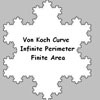### Von Koch Curve

##### Age 16 to 18 Challenge Level:

Make a poster using equilateral triangles with sides 27, 9, 3 and 1 units assembled as stage 3 of the Von Koch fractal. Investigate areas & lengths when you repeat a process infinitely often.### Sierpinski Triangle

##### Age 16 to 18 Challenge Level:

What is the total area of the triangles remaining in the nth stage of constructing a Sierpinski Triangle? Work out the dimension of this fractal.